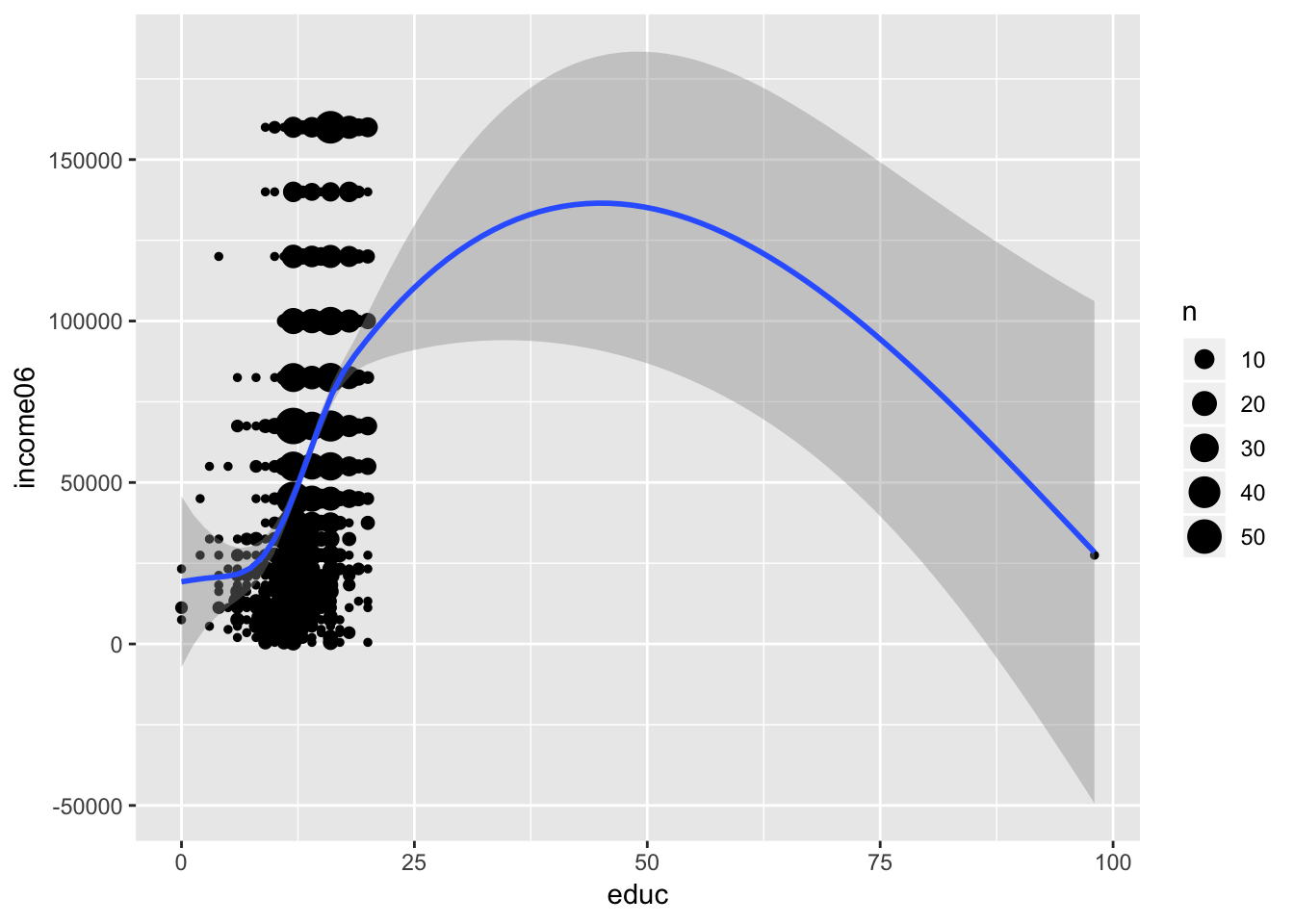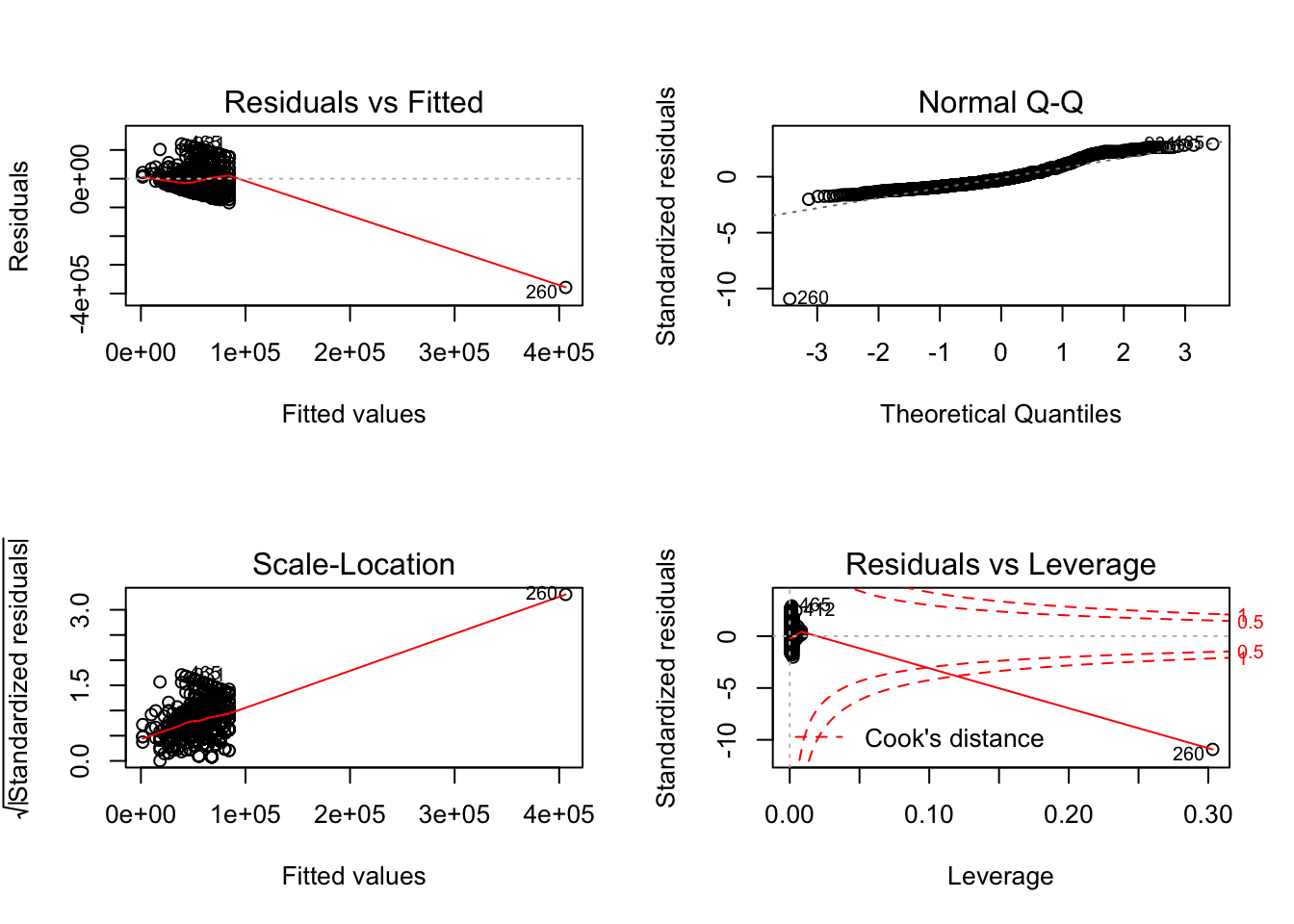## Introduction

Chapter 4 introduces statistical inference with regression. The following examples help illustrate a number of principles that were discussed. Follow all instructions to complete Chapter 4.

## Statistical Inference

1. Download the GSS_reduced_example.csv data set. Turns out that it is already saved for you in an R object in the educ7610 package. We can install this package (since it is on GitHub and not CRAN) with some wizardry.
# Run if you haven't installed remotes:
#   install.packages("remotes")
remotes::install_github("tysonstanley/educ7610")
1. Open RStudio, start a new R script or RMarkdown document.
2. Load the tidyverse package (you can ignore the notes that you see below that it gives you once you load it) and the furniture package.
library(tidyverse)
library(furniture)
library(educ7610)
1. Import it into R.
data(gss)
1. Use a simple regression model to assess the effect of the number of years of education (educ) on income (income06). Using summary() obtain the F-statistic of the model with it’s accompanying p-value, as well as the standard error, t-value, and p-value of the estimate itself.
gss %>%
lm(income06 ~ educ,
data = .) %>%
summary()
##
## Call:
## lm(formula = income06 ~ educ, data = .)
##
## Residuals:
##     Min      1Q  Median      3Q     Max
## -378667  -30013  -10390   20363  121117
##
## Coefficients:
##             Estimate Std. Error t value Pr(>|t|)
## (Intercept)   1741.9     3789.5    0.46    0.646
## educ          4126.8      270.4   15.26   <2e-16 ***
## ---
## Signif. codes:  0 '***' 0.001 '**' 0.01 '*' 0.05 '.' 0.1 ' ' 1
##
## Residual standard error: 41520 on 1772 degrees of freedom
##   (249 observations deleted due to missingness)
## Multiple R-squared:  0.1162, Adjusted R-squared:  0.1157
## F-statistic:   233 on 1 and 1772 DF,  p-value: < 2.2e-16
1. This output gives you the estimate, the standard error of the estimate, the t-value of the estimate, and the p-value of the estimate (where the null is that there is no relationship). Is the relationship statistically significant at the $$\alpha = .05$$ level?
2. From that same output above, we see a lot more information. What pieces do you recognize? Which pieces are confusing?
3. Let’s run a multiple regression with years of education (educ) on income (income06) while controlling for home population (hompop) and age (age).
gss %>%
lm(income06 ~ educ + hompop + age,
data = .) %>%
summary()
##
## Call:
## lm(formula = income06 ~ educ + hompop + age, data = .)
##
## Residuals:
##     Min      1Q  Median      3Q     Max
## -398142  -27672   -8912   19800  121471
##
## Coefficients:
##              Estimate Std. Error t value Pr(>|t|)
## (Intercept) -33491.96    5460.46  -6.134 1.06e-09 ***
## educ          4319.85     261.73  16.505  < 2e-16 ***
## hompop        8222.59     724.41  11.351  < 2e-16 ***
## age            251.22      59.52   4.221 2.56e-05 ***
## ---
## Signif. codes:  0 '***' 0.001 '**' 0.01 '*' 0.05 '.' 0.1 ' ' 1
##
## Residual standard error: 40110 on 1770 degrees of freedom
##   (249 observations deleted due to missingness)
## Multiple R-squared:  0.1762, Adjusted R-squared:  0.1748
## F-statistic: 126.2 on 3 and 1770 DF,  p-value: < 2.2e-16
1. How is the effect of education interpreted in this case? Is it statistically significant?
2. Let’s compare this to the regression value after we standardize both variables with scale(). We first have to grab just the complete cases of the variables first.
gss %>%
filter(complete.cases(income06, educ, hompop, age)) %>%
mutate(incomeZ = scale(income06) %>% as.numeric,
educZ   = scale(educ) %>% as.numeric,
hompopZ = scale(hompop) %>% as.numeric,
ageZ    = scale(age) %>% as.numeric) %>%
lm(incomeZ ~ educZ + hompopZ + ageZ,
data = .) %>%
summary()
##
## Call:
## lm(formula = incomeZ ~ educZ + hompopZ + ageZ, data = .)
##
## Residuals:
##     Min      1Q  Median      3Q     Max
## -9.0181 -0.6268 -0.2019  0.4485  2.7514
##
## Coefficients:
##               Estimate Std. Error t value Pr(>|t|)
## (Intercept) -1.196e-16  2.157e-02   0.000        1
## educZ        3.568e-01  2.162e-02  16.505  < 2e-16 ***
## hompopZ      2.628e-01  2.315e-02  11.351  < 2e-16 ***
## ageZ         9.756e-02  2.311e-02   4.221 2.56e-05 ***
## ---
## Signif. codes:  0 '***' 0.001 '**' 0.01 '*' 0.05 '.' 0.1 ' ' 1
##
## Residual standard error: 0.9084 on 1770 degrees of freedom
## Multiple R-squared:  0.1762, Adjusted R-squared:  0.1748
## F-statistic: 126.2 on 3 and 1770 DF,  p-value: < 2.2e-16
1. Is the standardized estimates more/less/same significant as the unstandardized? Is this surprising?
2. We can also test the significance of a predictor using model comparisons. Let’s look at adding educ to the null model (a model with just an intercept). We’ll use anova() to compare the two models. Does the result match what we saw earlier?
fit_null <- gss %>%
lm(income06 ~ 1,
data = .)
fit_educ <- gss %>%
lm(income06 ~ educ,
data = .)
anova(fit_null, fit_educ)
## Analysis of Variance Table
##
## Model 1: income06 ~ 1
## Model 2: income06 ~ educ
##   Res.Df        RSS Df Sum of Sq   F    Pr(>F)
## 1   1773 3.4558e+12
## 2   1772 3.0542e+12  1 4.016e+11 233 < 2.2e-16 ***
## ---
## Signif. codes:  0 '***' 0.001 '**' 0.01 '*' 0.05 '.' 0.1 ' ' 1

## Check Some Assumptions

For inference to be meaningful at all, we need to check if our assumptions are holding. As a reminder, for a linear regression model we have 4 main assumptions:

• Linearity
• Homoscedasticity
• Conditional Distribution of Y is normal and centered at $$\hat{Y}$$
• Independent Sampling
1. Let’s test these assumptions using plots as we discussed in class. First, let’s see if the relationship between income06 and educ is linear. This one we can take a look at via a scatterplot with a smoothed line showing the relationship. (Note: due to overplotting–points on top of points–we used geom_count() instead of geom_point().)
gss %>%
ggplot(aes(educ, income06)) +
geom_count() +
geom_smooth()1. That scatterplot does not look good because there is an outlier there. This is an example of one of the many reasons we want to visualize our data before modeling it. That point is likely problematic (it is a high leverage point – we’ll be talking about this later on).
2. Next let’s look at homoscedasticity. This we will look at using our model object. Using the plot() function with our model object we get four plots. The first one let’s us assess our residuals for homoscedasticity. Does it look homoscedastic?
fit_educ <- gss %>%
lm(income06 ~ educ,
data = .)
par(mfrow = c(2,2))
plot(fit_educ)1. The second plot above shows us whether our residuals are approximately normal. The others we’ll get to later. Do the residuals follow the line or do they deviate?
2. Finally, independent sampling is not an assumption that we can check. This is something that has to be taken care of during data collection; not during the analysis. So for this one, we will assume the data were collected in this fashion.
3. Because of the outlier, everything was somewhat thrown off. Let’s take that outlier out and try these again.
4. Let’s test these assumptions using plots as we discussed in class. First, let’s see if the relationship between income06 and educ is linear. This one we can take a look at via a scatterplot. Does it look linear? (Note: due to overplotting–points on top of points–we used geom_count() instead of geom_point().)
gss %>%
mutate(educ = furniture::washer(educ, 99, 98)) %>%   ## removes all 99's from educ
ggplot(aes(educ, income06)) +
geom_count() +
geom_smooth()